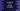# How to get all Keys of a JavaScript Object### Introduction:

JavaScript objects hold key-value pairs. There are different ways to get only the keys of an Object. We can either iterate through the keys of an object or we can use the method Object.keys() to get them.

Let’s learn these methods with examples.

### Method 1: By using Object.keys():

The Object.keys() method returns an array of all the enumerable property names of an object. It has the following syntax:

``Object.keys(o)``

Where o is the object to find the keys.

It will return an array of strings, that represents the enumerable properties of the given object, i.e. it will return an array of keys of that object.

For example, let’s take a look at the below example program:

``````let givenObject = { name: "Alex", age: 20 };

let keys = Object.keys(givenObject);

console.log(keys);``````

In this example, we are using Object.keys method to get the keys of givenObject. If you run this, it will print:

``[ 'name', 'age' ]``

### Method 2: By iterating through the keys of an Object:

We can always iterate through the keys of an object and add them to an array. We have to follow the following steps:

• Initialize an empty array to hold the keys
• Iterate through the keys of the object by using a loop
• Add the keys to the array.
• Print the array.
``````let givenObject = { name: "Alex", age: 20 };

let keys = [];

for(let k in givenObject){
keys.push(k);
}

console.log(keys);``````

Here, keys is the empty array initialized at the start of the program. The for-in loop iterates through the keys of the object givenObject and we are adding them to the array.

It will print the same output.

### Get all values of an object:

We can also get the values of an object. We have to use Object.values to get these:

``````let givenObject = { name: "Alex", age: 20 };

let v = Object.values(givenObject);

console.log(v);``````

It will print the values of givenObject.

``[ 'Alex', 20 ]``

#### Reference:

https://developer.mozilla.org/en-US/docs/Web/JavaScript/Reference/Global_Objects/Object/keys